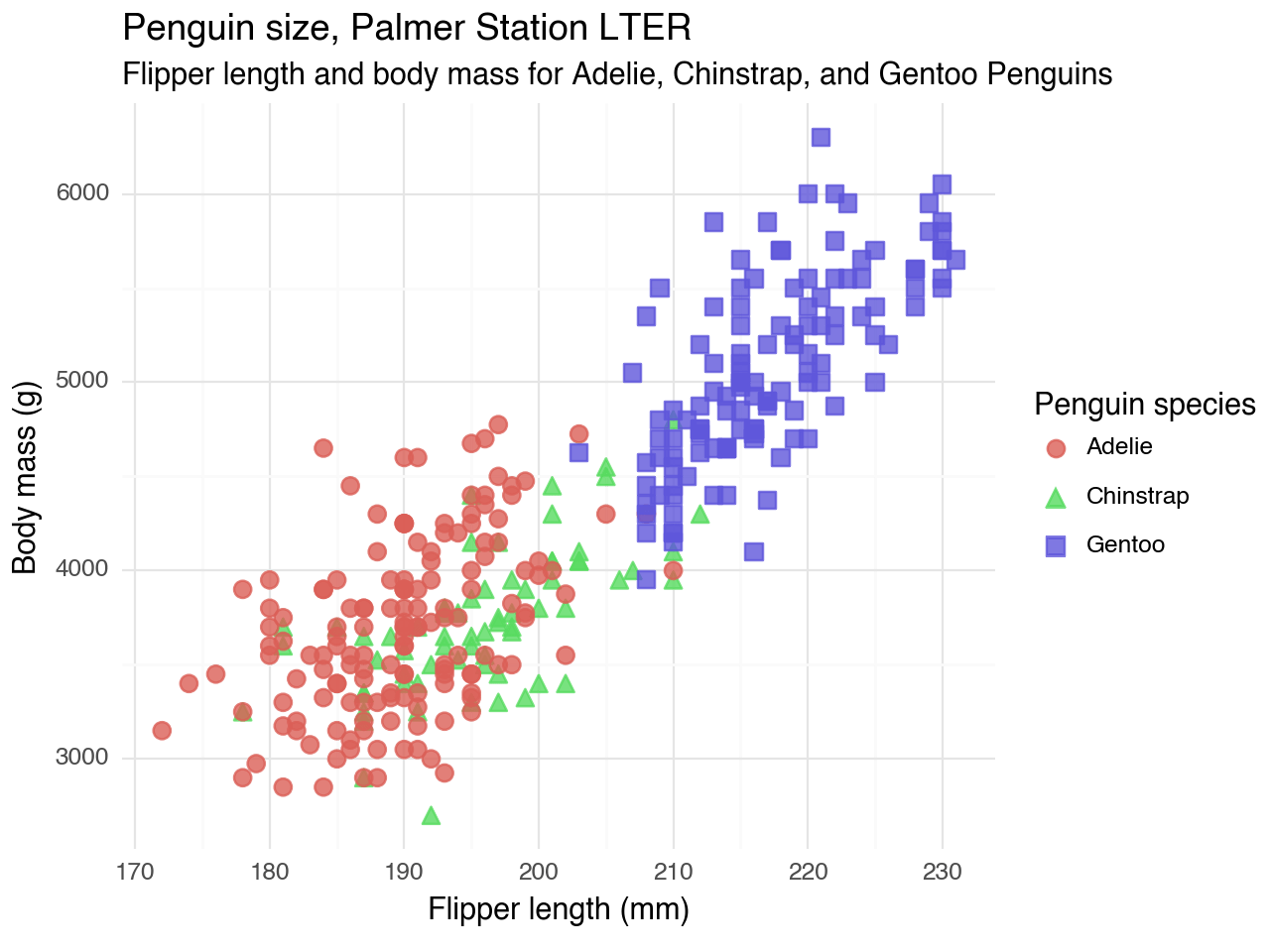Want to share your content on R-bloggers? click here if you have a blog, or here if you don't.

As I’ve been saying every year for the past seven years or so, I am learning Python. (It’s been a journey.)

Python packages like pandas have several ways to work with data. There are several options for indexing, slicing, etc. They have a lot of flexibility but also a lot of conventions to remember.

I am familiar with the grammar of the tidyverse, which provides a consistent set of verbs to solve common data manipulation challenges. I investigated ways to port tidyverse-like verbs to Python (hopefully making Python a little easier to grasp).

Here are three packages that do just that.

## siuba

The siuba package, created by Michael Chow, allows you to use dplyr-like syntax with pandas. Siuba ports over several functions, including `select()`, `filter()`, `mutate()`, `summarize()`, and `arrange()`. The package also allows you to use `group_by()` and a `>>` pipe.

Let’s check out a few examples using the palmerpenguins dataset (R, Python).

```from palmerpenguins import load_penguins
from siuba import group_by, summarize, _

(penguins
>> group_by(_.species)
>> summarize(n = _.species.count())
)```
species n
1 Chinstrap 68
2 Gentoo 124
```library(palmerpenguins)
library(dplyr)

(penguins %>%
group_by(species) %>%
summarize(n = n()))
# A tibble: 3 × 2
species       n
<fct>     <int>
2 Chinstrap    68
3 Gentoo      124```

Thanks to the documentation and interactive tutorials available for siuba, it’s easy to see the parallels and differences with dplyr so that you can craft these functions yourself.

```from palmerpenguins import load_penguins
from siuba import select

(penguins
>> select(-_.isalpha(), _.species)
>> group_by(_.species)
>> summarize(
bill_length_mm = _.bill_length_mm.mean(),
bill_depth_mm = _.bill_depth_mm.mean(),
flipper_length_mm = _.flipper_length_mm.mean(),
body_mass_g = _.body_mass_g.mean()
)
)```
species bill_length_mm bill_depth_mm flipper_length_mm body_mass_g
0 Adelie 38.791391 18.346358 189.953642 3700.662252
1 Chinstrap 48.833824 18.420588 195.823529 3733.088235
2 Gentoo 47.504878 14.982114 217.186992 5076.016260
```(penguins %>%
group_by(species) %>%
summarize(across(!where(is.character), mean, na.rm = TRUE)))
# A tibble: 3 × 8
species   island bill_length_mm bill_depth_mm flipper_length_mm body_mass_g
<fct>      <dbl>          <dbl>         <dbl>             <dbl>       <dbl>
1 Adelie        NA           38.8          18.3              190.       3701.
2 Chinstrap     NA           48.8          18.4              196.       3733.
3 Gentoo        NA           47.5          15.0              217.       5076.
# … with 2 more variables: sex <dbl>, year <dbl>```

## plotnine

The plotnine package, created by Hassan Kibirige, lets you use a grammar of graphics for Python.

You can use siuba and plotnine together, similar to how you would use dplyr and ggplot2 together.

```from siuba import *
from plotnine import *

(penguins
# using siuba pipe
>> ggplot(aes(x = 'flipper_length_mm', y = 'body_mass_g'))
# creating plotnine plot
+ geom_point(aes(color = 'species', shape = 'species'),
size = 3,
alpha = 0.8)
+ theme_minimal()
+ labs(title = "Penguin size, Palmer Station LTER",
subtitle = "Flipper length and body mass for Adelie, Chinstrap, and Gentoo Penguins",
x = "Flipper length (mm)",
y = "Body mass (g)",
color = "Penguin species",
shape = "Penguin species"))````<ggplot: (326970103)>`

Folks have heuristics to translate ggplot2 code to plotnine. These help understand the nuances between the two.

## pyjanitor

OK, this one is cheating a bit because the janitor package by Sam Firke is not part of the tidyverse. One more package that uses ‘tidyverse-like’ verbs is pyjanitor. With pyjanitor, you can clean column names, identify duplicate entries, and more.

```from janitor import clean_names
import pandas as pd
import numpy as np

example_df = {
'Terrible Name 1': ['name1', 'name2', 'name3', 'name4'],
'PascalCase': [150.0, 200.0, 300.0, 400.0],
'this_has_punctuation?': [np.nan, np.nan, np.nan, np.nan],
}

pd.DataFrame.from_dict(example_df).clean_names()```
terrible_name_1 pascalcase this_has_punctuation_
0 name1 150.0 NaN
1 name2 200.0 NaN
2 name3 300.0 NaN
3 name4 400.0 NaN

Python’s pandas allows you to method chain with pipes. They can be used with pyjanitor, as well.

```from janitor import clean_names, remove_empty
import pandas as pd
import numpy as np

example_df = {
'Terrible Name 1': ['name1', 'name2', 'name3', 'name4'],
'PascalCase': [150.0, 200.0, 300.0, 400.0],
'this_has_punctuation?': [np.nan, np.nan, np.nan, np.nan],
}

(pd.DataFrame.from_dict(example_df)
.pipe(clean_names)
.pipe(remove_empty)
)```
terrible_name_1 pascalcase
0 name1 150.0
1 name2 200.0
2 name3 300.0
3 name4 400.0

## Conclusion

While Python syntax and conventions are still on my “to-learn” list, it is helpful to know there are packages that can bring the familiarity of the tidyverse to Python.